# CHSE Odisha Class 12 Math Solutions Chapter 4 Matrices Ex 4(a)

Odisha State Board Elements of Mathematics Class 12 CHSE Odisha Solutions Chapter 4 Matrices Ex 4(a) Textbook Exercise Questions and Answers.

## CHSE Odisha Class 12 Math Solutions Chapter 4 Matrices Exercise 4(a)

Question 1.
State the order of the following matrices.
(i) [abc]
(ii) $$\left[\begin{array}{l} 1 \\ 2 \end{array}\right]$$
(iii) $$\left[\begin{array}{ll} x & y \\ y & z \\ z & x \end{array}\right]$$
(iv) $$\left[\begin{array}{cccc} 1 & 0 & 1 & 4 \\ 2 & 1 & 3 & 0 \\ -3 & 2 & 1 & 3 \end{array}\right]$$
Solution:
(i) (1 x 3)
(ii) (2 x 1)
(iii) (3 x 2)
(iv) (3 x 4)

Question 2.
How many entries are there in a
(i) 3 x 3 matrix
(ii) 3 x 4 matrix
(iii) p x q matrix
(iv) a sqare matrix of order p?
Solution:
(i) 9
(ii) 12
(iii) pq
(iv) p2Question 3.
Give an example of
(i) 3 x 1 matrix
(ii) 2 x 2 matrix
(iii) 4 x 2 matrix
(iv) 1 x 3 matrix
Solution:
(i) $$\left(\begin{array}{l} a \\ b \\ c \end{array}\right)$$
(ii) $$\left(\begin{array}{ll} a & b \\ c & d \end{array}\right)$$
(iii) $$\left(\begin{array}{ll} a & b \\ c & d \\ e & f \\ g & h \end{array}\right)$$
(iv) (1, 2, 3)

Question 4.
Let A = $$\left[\begin{array}{lllll} 1 & 2 & 3 & 4 & 1 \\ 4 & 5 & 6 & 1 & 2 \\ 3 & 9 & 1 & 1 & 6 \end{array}\right]$$
(i) What is the order of A?
(ii) Write down the entries a31, a25, a23
(iii) Write down AT.
(iv) What is the order of AT?
Solution:
A = $$\left[\begin{array}{lllll} 1 & 2 & 3 & 4 & 1 \\ 4 & 5 & 6 & 1 & 2 \\ 3 & 9 & 1 & 1 & 6 \end{array}\right]$$
(i) Order of A is (3 x 5)
(ii) a31 = 3, a25= 2, a23 = 6
(iii) AT = $$\left[\begin{array}{lll} 1 & 4 & 3 \\ 2 & 5 & 9 \\ 3 & 6 & 1 \\ 4 & 1 & 1 \\ 1 & 2 & 6 \end{array}\right]$$
(iv) Order of AT is (5 x 3).

Question 5.
Matrices A and B are given below. Find A + B, B + A, A – B and B – A. Verify that A + B = B + A and B – A = -(A – B)
(i) A = $$\left[\begin{array}{l} 7 \\ 1 \end{array}\right]$$, B = $$\left[\begin{array}{c} -6 \\ 9 \end{array}\right]$$
Solution: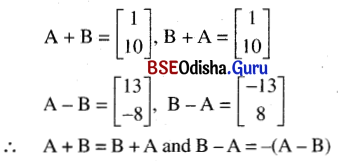(ii) A = $$\left[\begin{array}{cc} 1 & 2 \\ 3 & -1 \end{array}\right]$$, B = $$\left[\begin{array}{cc} 4 & 1 \\ -3 & 2 \end{array}\right]$$
Solution: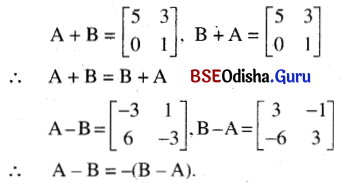(iii) A = $$\left[\begin{array}{ll} \frac{1}{2} & \frac{1}{4} \\ \frac{1}{3} & \frac{1}{5} \end{array}\right]$$, B = $$\left[\begin{array}{ll} \frac{1}{3} & \frac{1}{2} \\ \frac{1}{2} & \frac{4}{5} \end{array}\right]$$
Solution: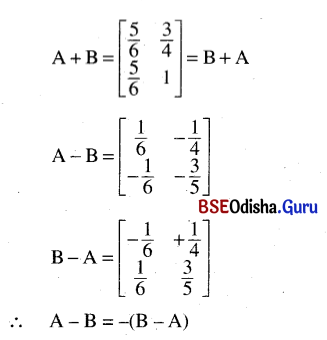(iv) A = $$\left[\begin{array}{cc} 1 & a-b \\ a+b & -3 \end{array}\right]$$, B = $$\left[\begin{array}{cc} 1 & b \\ -a & 5 \end{array}\right]$$
Solution: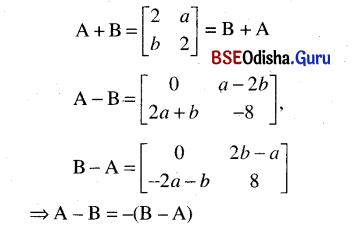(v) $$\left[\begin{array}{rrr} 1 & -2 & 5 \\ -1 & 4 & 3 \\ 1 & 2 & -3 \end{array}\right]$$, B = $$\left[\begin{array}{rrr} -1 & 2 & -5 \\ 1 & -3 & -3 \\ 1 & 2 & 4 \end{array}\right]$$
Solution: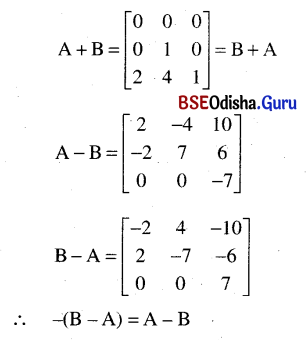Question 6.
(i) Find the 2×2 matrix X
if X + $$\left[\begin{array}{ll} 0 & 1 \\ 1 & 0 \end{array}\right]$$ = $$\left[\begin{array}{ll} 2 & 0 \\ 0 & 2 \end{array}\right]$$
Solution: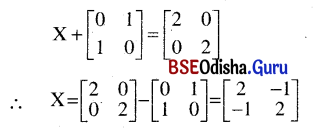(ii) Given
[x y z] – [-4 3 1] = [-5 1 0] derermine x, y, z.
Solution:
[x y z] – [-4 3 1] = [-5 1 0]
∴ (x y z) = (-4 3 1) + (-5 1 0) = (-9 4 1)
∴ x = -9, y = 4, z = 1

(iii) If $$\left[\begin{array}{ll} x_1 & x_2 \\ y_1 & y_2 \end{array}\right]$$ – $$\left[\begin{array}{ll} 2 & 3 \\ 0 & 1 \end{array}\right]$$ = $$\left[\begin{array}{ll} 3 & 5 \\ 1 & 2 \end{array}\right]$$ determine x1, x2, y1, y2.
Solution: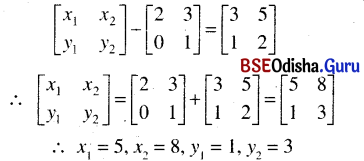(iv) Find a matrix which when added to $$\left[\begin{array}{cc} 2 & -3 \\ -4 & 7 \end{array}\right]$$ gives $$\left[\begin{array}{ll} 4 & 1 \\ 3 & 2 \end{array}\right]$$
Solution: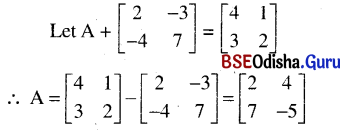Question 7.
Calculate whenever possible, the following products.
(i) $$\left[\begin{array}{ll} 1 & 2 \\ 3 & 4 \end{array}\right]\left[\begin{array}{l} 2 \\ 3 \end{array}\right]$$
Solution: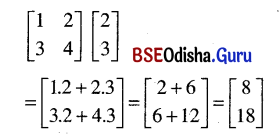(ii) $$\left[\begin{array}{l} 2 \\ 3 \end{array}\right]\left[\begin{array}{ll} 1 & 2 \\ 4 & 3 \end{array}\right]$$
Solution:
$$\left[\begin{array}{l} 2 \\ 3 \end{array}\right]\left[\begin{array}{ll} 1 & 2 \\ 4 & 3 \end{array}\right]$$ is impossible because number of columns of 1st ≠ number of rows of second.

(iii) $$\left[\begin{array}{ll} 1 & 2 \\ 2 & 1 \end{array}\right]\left[\begin{array}{ll} 3 & 1 \\ 1 & 1 \end{array}\right]$$
Solution: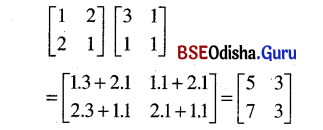(iv) $$\left[\begin{array}{cc} 1 & -2 \\ -2 & 3 \end{array}\right]\left[\begin{array}{lll} 1 & 2 & 3 \\ 2 & 3 & 4 \end{array}\right]$$
Solution: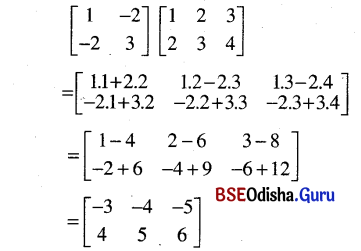Question 8.
If A = $$\left[\begin{array}{ll} 1 & 2 \\ 3 & 4 \end{array}\right]$$, B = $$\left[\begin{array}{ll} 3 & 2 \\ 1 & 4 \end{array}\right]$$, C = $$\left[\begin{array}{ll} 2 & 2 \\ 1 & 3 \end{array}\right]$$
Calculate (i) AB (ii) BA (iii) BC (iv) CB (v) AC (vi) CA
Solution: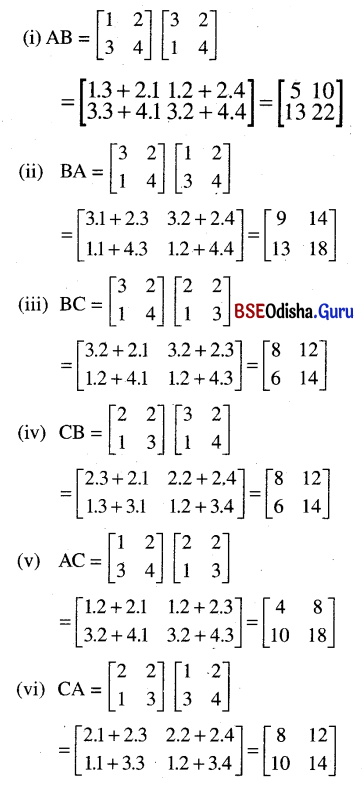Question 9.
Find the following products.
(i) $$\left[\begin{array}{ll} 1 & 2 \\ 3 & 4 \end{array}\right]\left[\begin{array}{ll} 1 & 0 \\ 0 & 1 \end{array}\right]$$
Solution: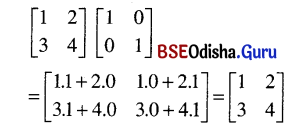(ii) $$\left[\begin{array}{ll} 1 & 0 \\ 0 & 1 \end{array}\right]\left[\begin{array}{ll} 1 & 2 \\ 3 & 4 \end{array}\right]$$
Solution: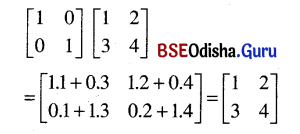(iii) $$\left[\begin{array}{ll} 1 & 2 \\ 3 & 4 \end{array}\right]\left[\begin{array}{ll} 1 & 3 \\ 1 & 4 \end{array}\right]$$
Solution: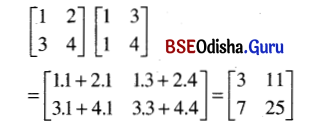(iv) $$\left[\begin{array}{ll} 1 & 3 \\ 1 & 4 \end{array}\right]\left[\begin{array}{ll} 1 & 2 \\ 3 & 4 \end{array}\right]$$
Solution: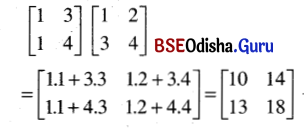(v) $$\left[\begin{array}{cc} 1 & i \\ i & -1 \end{array}\right]^2$$ where i = √-1
Solution: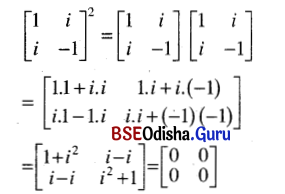(vi) $$\left[\begin{array}{ll} \mathbf{0} & \mathbf{1} \\ \mathbf{1} & \mathbf{0} \end{array}\right]\left[\begin{array}{ll} a & b \\ c & d \end{array}\right]$$
Solution: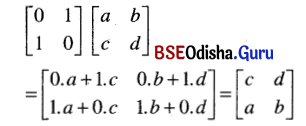(vii) $$\left[\begin{array}{ll} 0 & k \\ 1 & 0 \end{array}\right]\left[\begin{array}{ll} a & b \\ c & d \end{array}\right]$$
Solution: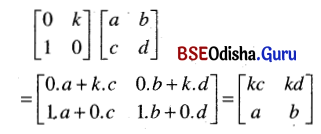(viii) $$\left[\begin{array}{ll} a & b \\ c & d \end{array}\right]\left[\begin{array}{ll} 0 & 1 \\ 1 & 0 \end{array}\right]$$
Solution: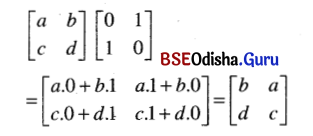(ix) $$\left[\begin{array}{ll} 1 & 0 \\ 0 & k \end{array}\right]\left[\begin{array}{ll} a & b \\ c & d \end{array}\right]$$
Solution: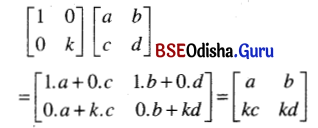(x) $$\left[\begin{array}{lll} 1 & 2 & 3 \\ 4 & 5 & 6 \\ 7 & 8 & 9 \end{array}\right]\left[\begin{array}{lll} 0 & 0 & 0 \\ 0 & 0 & 0 \\ 0 & 0 & 0 \end{array}\right]$$
Solution:
$$\left[\begin{array}{lll} 1 & 2 & 3 \\ 4 & 5 & 6 \\ 7 & 8 & 9 \end{array}\right]\left[\begin{array}{lll} 0 & 0 & 0 \\ 0 & 0 & 0 \\ 0 & 0 & 0 \end{array}\right]$$ = $$\left[\begin{array}{lll} 0 & 0 & 0 \\ 0 & 0 & 0 \\ 0 & 0 & 0 \end{array}\right]$$Question 10.
Write true or false in the following cases:
(i) The sum of a 3 x 4 matrix with a 3 x 4 matrix is a 3 x 3 matrix.
Solution:
False

(ii) k = 0, k ∈ R
Solution:
False

(iii) A – B = B – A, if one of A and B is zero and A and B are of the same order.
Solution:
False

(iv) A + B = B + A, if A and B are matrices of the same order.
Solution:
True

(v) $$\left[\begin{array}{cc} 1 & 0 \\ -2 & 0 \end{array}\right]$$ + $$\left[\begin{array}{cc} -1 & 0 \\ 2 & 0 \end{array}\right]$$ = 0
Solution:
True

(vi) $$\left[\begin{array}{ll} 3 & 1 \\ 6 & 2 \end{array}\right]$$ = 3 $$\left[\begin{array}{ll} 1 & 1 \\ 2 & 2 \end{array}\right]$$
Solution:
False

(vii) With five elements a matrix can not be constructed.
Solution:
False

(viii)The unit matrix is its own transpose.
Solution:
True

Question 11.
If A = $$\left[\begin{array}{cc} 2 & 4 \\ 3 & 13 \end{array}\right]$$ and I = $$\left[\begin{array}{ll} 1 & 0 \\ 0 & 1 \end{array}\right]$$ find A – α I, α ∈ R.
Solution: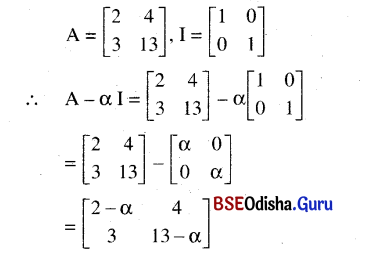Question 12.
Find x and y in the following.
(i) $$\left[\begin{array}{cc} x & -2 y \\ 0 & -2 \end{array}\right]=\left[\begin{array}{cc} 1 & -8 \\ 0 & -2 \end{array}\right]$$
Solution: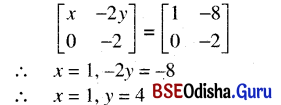(ii) $$\left[\begin{array}{c} x+3 \\ 2-y \end{array}\right]=\left[\begin{array}{c} 1 \\ -3 \end{array}\right]$$
Solution: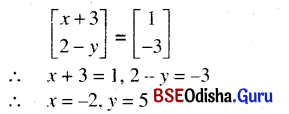(iii) $$\left[\begin{array}{c} 2 x-y \\ x+y \end{array}\right]=\left[\begin{array}{c} 3 \\ -9 \end{array}\right]$$
Solution: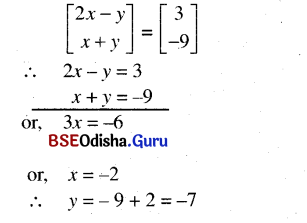(iv) $$\left[\begin{array}{l} x \\ y \end{array}\right]+\left[\begin{array}{l} 3 \\ 4 \end{array}\right]=\left[\begin{array}{c} 2 \\ -1 \end{array}\right]$$
Solution: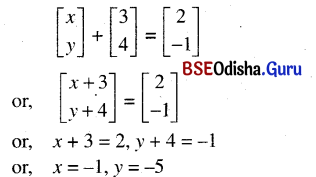(v) [2x -y] + [y 3x] = 5 [1 0]
Solution: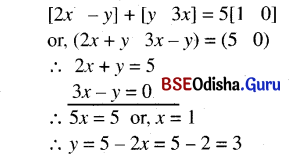Question 13.
The element of ith row and ith column of the following matrix is i +j. Complete the matrix.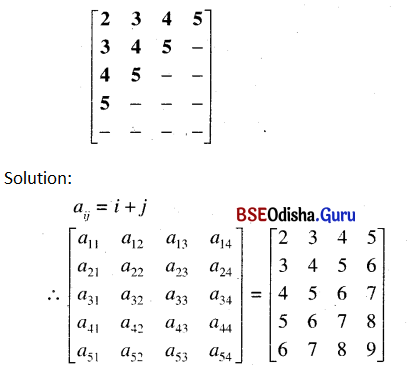Question 14.
Write down the matrix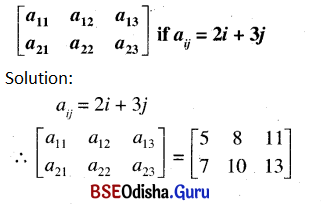Question 15.
Construct a 2 x 3 matrix having elements given by
(i) aij = i + j
(ii) aij = i – j
(iii) aij = i × j
(iv) aij = i / j
Solution: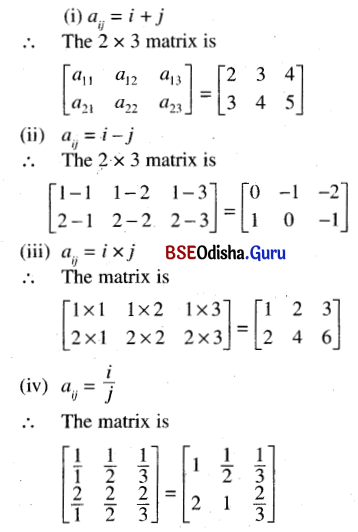Question 16.
If $$\left[\begin{array}{cc} 2 x & y \\ 1 & 3 \end{array}\right]+\left[\begin{array}{cc} 4 & 2 \\ 0 & -1 \end{array}\right]=\left[\begin{array}{ll} 8 & 3 \\ 1 & 2 \end{array}\right]$$
Solution: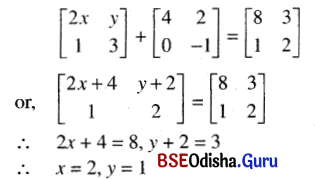Question 17.
Find A such that
$$\left[\begin{array}{ccc} 2 & 3 & 4 \\ 1 & 0 & -2 \\ 3 & 1 & -1 \end{array}\right]+A=\left[\begin{array}{ccc} 1 & 2 & -1 \\ 2 & -1 & 0 \\ 1 & 3 & 2 \end{array}\right]$$
Solution: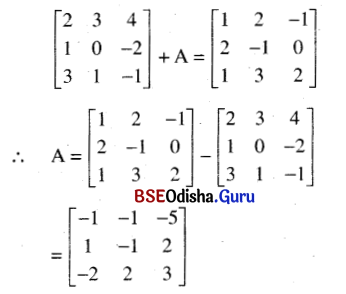Question 18.
If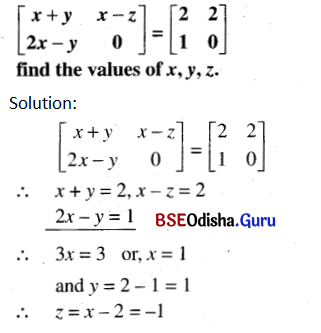Question 19.
What is the order of the matrix B if [3 4 2] B = [2 1 0 3 6]
Solution:
(3 4 2) B = (2 1 0 3 6)
Let A = (3 4 2), C = (2 1 0 3 6)
∴ Order of A = (1 x 3)
Order of C = (1 x 5)
∴ Order of B = (3 x 5)

Question 20.
Find A if $$\left[\begin{array}{l} 4 \\ 1 \\ 3 \end{array}\right]$$ A = $$\left[\begin{array}{rrr} -4 & 8 & 4 \\ -1 & 2 & 1 \\ -3 & 6 & 3 \end{array}\right]$$
Solution: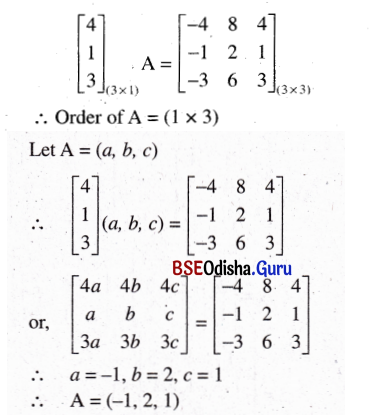Question 21.
Find B if B2 = $$\left[\begin{array}{cc} 17 & 8 \\ 8 & 17 \end{array}\right]$$
Solution: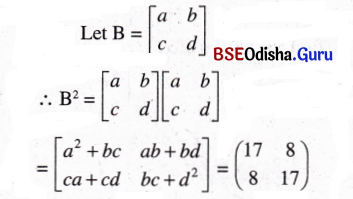∴ a2 + bc = 17, ab + bd= 8
ca + cd = 8, bc + d2 = 17
∴ a2 + bc = bc + d2
or, a2 + d2 or, a = d
or, ca + cd = ab + bd
or, cd + cd – bd + bd
or, 2cd = 2bd = 8
or, b = c and bd = 4 = cd
∴ ab + bd= 8
or, ab + 4 = 8
or, ab = 4
Again, a2 + bc = 17
or, a2 + b . b = 17 (b = c)
or, a2 + b2 = 17
Also (a + b)2 = a2 + b2 + 2ab
∴ (a + b)2 = 17 + 8 = 25
or, a + b = 5
And (a – b)2 = 17 – 8 = 9
or, a – b = 3
∴ a = 4, b = 1, So d = 4, c = 1
∴ B = $$\left[\begin{array}{ll} 4 & 1 \\ 1 & 4 \end{array}\right]$$Question 22.
Find x and y when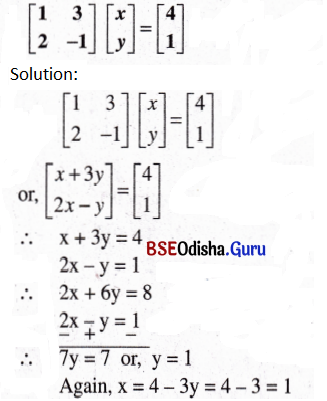Question 23.
Find AB and BA given that: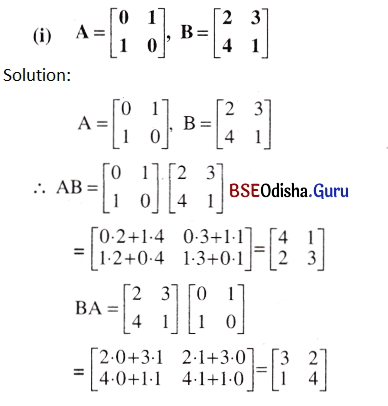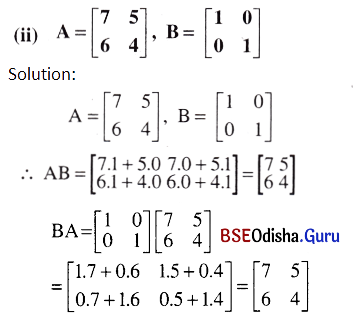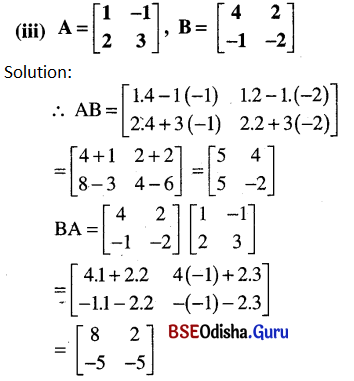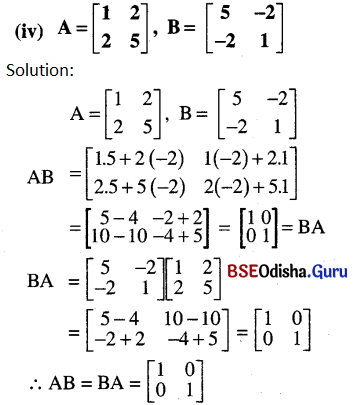Question 24.
Evaluate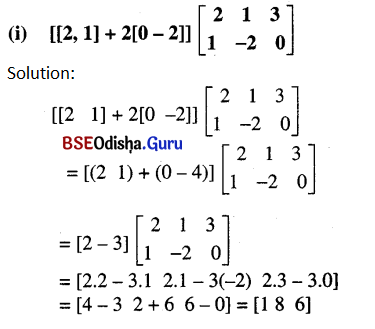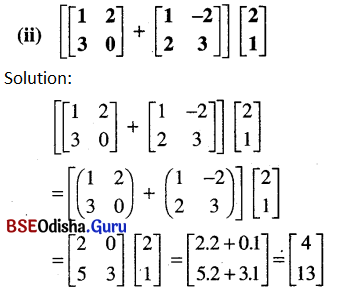Question 25.
If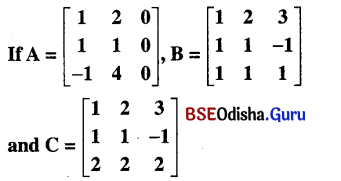Show that AB = AC though B ≠ C. Verify that
(i) A + (B + C) = (A + B) + C
(ii) A(B + C) = AB + AC
(iii) A(BC) = (AB)C
Solution: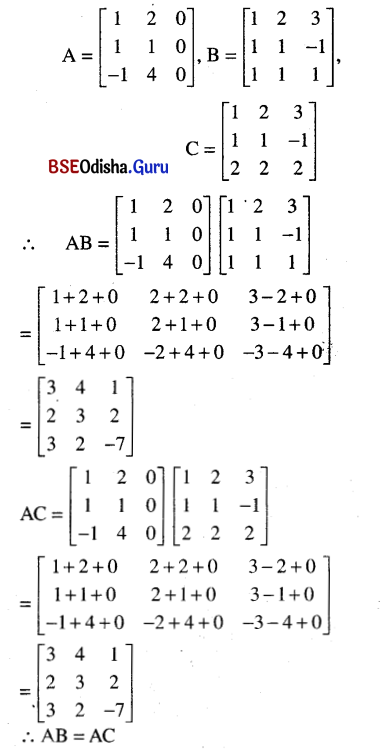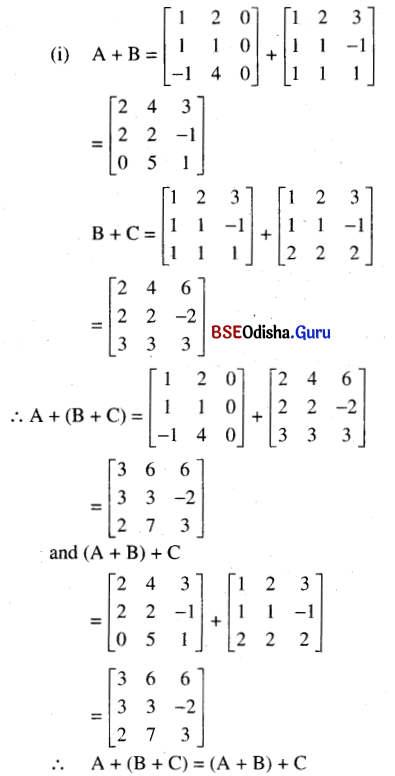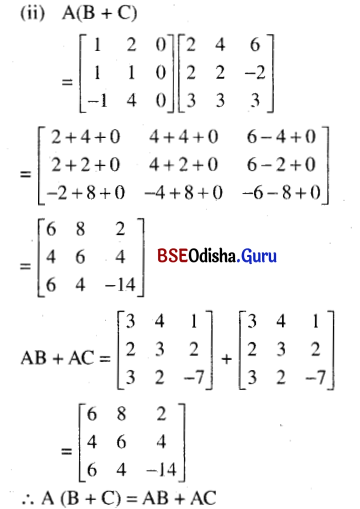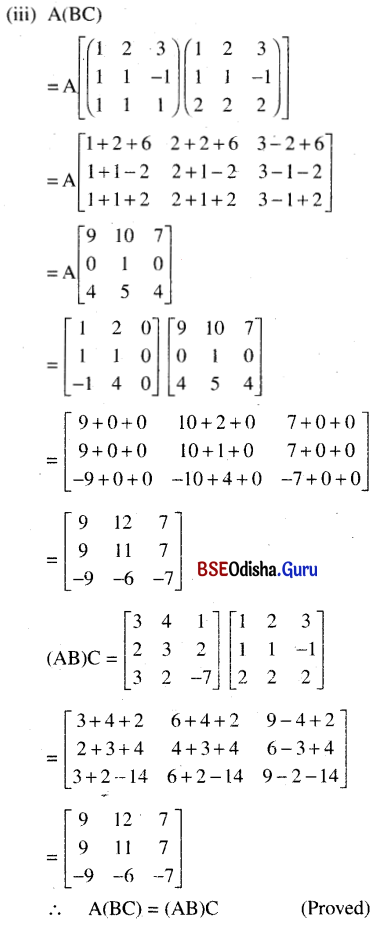Question 26.
Find A and B where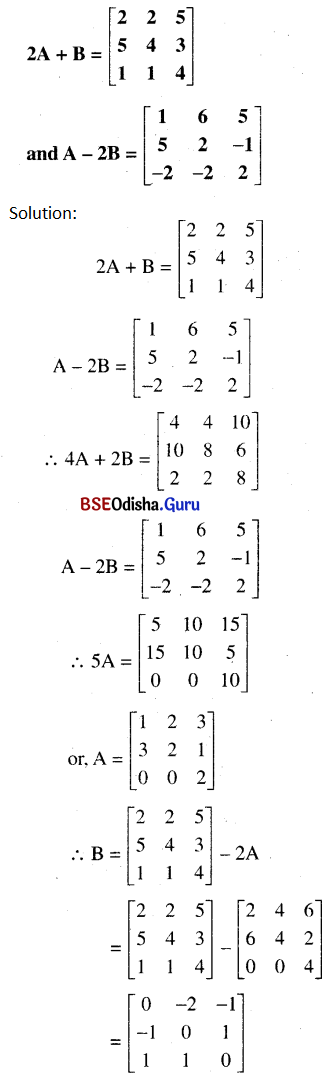Question 27.
If A = $$\left[\begin{array}{cc} 4 & 2 \\ -1 & 1 \end{array}\right]$$ and I be the 2 × 2 unit matrix find (A – 2I) (A – 3I)
Solution: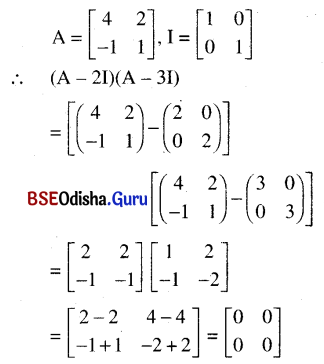Question 28.
Verify that [AB]T = BTAT where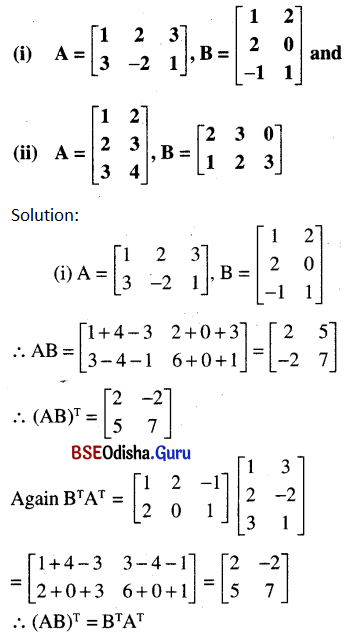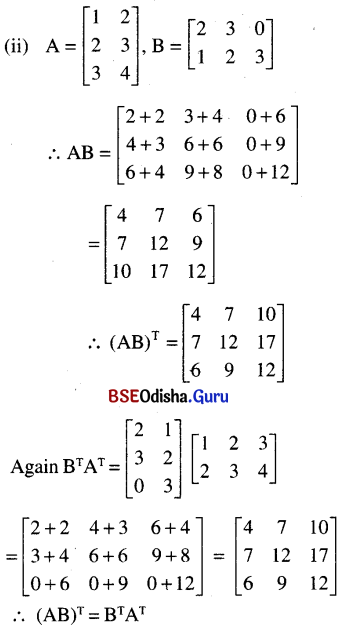Question 29.
Verify that A = $$\left[\begin{array}{ll} a & b \\ c & d \end{array}\right]$$ satisfies the equation x2 – (a + d)x + (ad – bc)I = 0 where I is the 2 x 2 matrix.
Solution: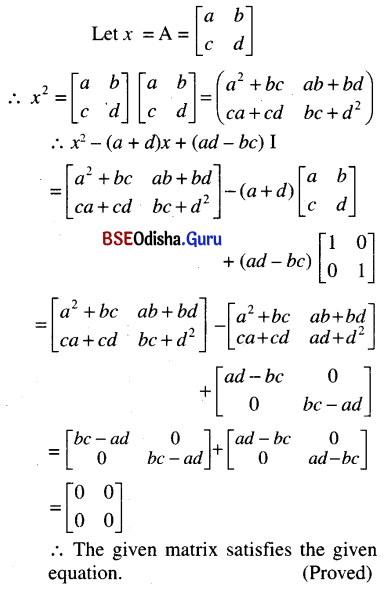Question 30.
If A = $$\left[\begin{array}{rrr} 1 & 2 & 3 \\ 3 & -2 & 1 \\ 4 & 2 & 1 \end{array}\right]$$, show that A3 – 23 A – 40 I = 0
Solution: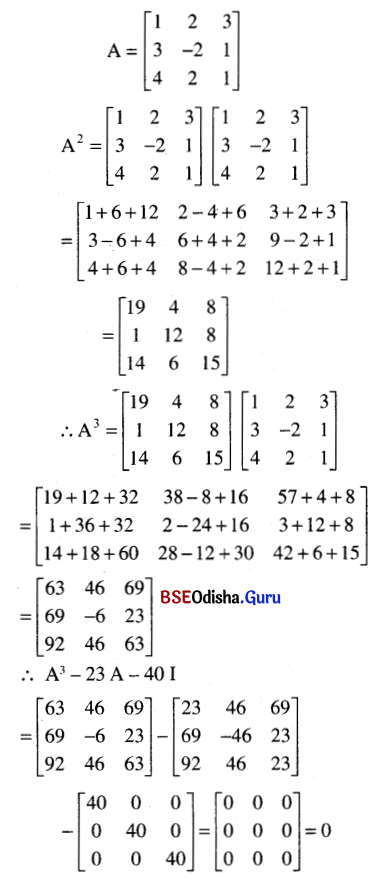Question 31.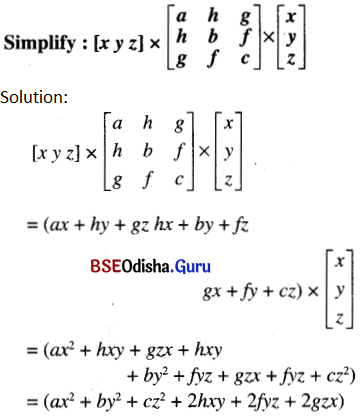Question 32.
If A and B are matrices of the same order and AB = BA, then prove that
(i) A2 – B2 = (A – B) (A + B)
(ii) A2 + 2AB + B2 = (A + B)2
(iii) A2 – 2AB + B2 = (A – B)2
Solution:
(i) (A – B) (A + B)
= A2 + AB – BA – B2
= A2 + AB – AB- B2( AB = BA)
= A2 – B2
(ii) (A + B)2 = (A + B) (A + B)
= A2 + AB + BA + B2
= A2 + AB + AB + B2 ( AB = BA)
= A2 + 2AB + B2
(iii) (A – B)2 = (A – B) (A – B)
= A2 – AB – BA + B2
= A2 – AB – AB + B2 (AB = BA)
= A2 – 2AB + B2

Question 33.
If α and β are scalars and A is a square matrix then prove that
(A – αI) . (A – βI) = A2 – (α + β) A + αβI, where I is a unit matrix of same order as A.
Solution:
(A – αI) (A – βI)
= A2 – AβI – αIA + αβI2
= A2 – βAI – αA + αβI
( IA = A, I2 = I)
= A2 – βA – αA + αβI) ( AI = A)
= A2 – (α + β) A + αβI

Question 34.
If α and β are scalars such that A = αβ + βI, where A, B and the unit matrix I are of the same order, then prove that AB = BA.
Solution:
We have A = αβ + βI
AB (αβ + βI) B
= α βB + βI B
= α βB + βB = (α + I) βB
= βB (α + 1)
( Scalar mltiβlication is associative)
= Bβ (α + 1)
= Bβα + Bβ = Bαβ + BIβ
( BI = B)
= B (αβ + βi) = BA
AB = BA
(proved)Question 35.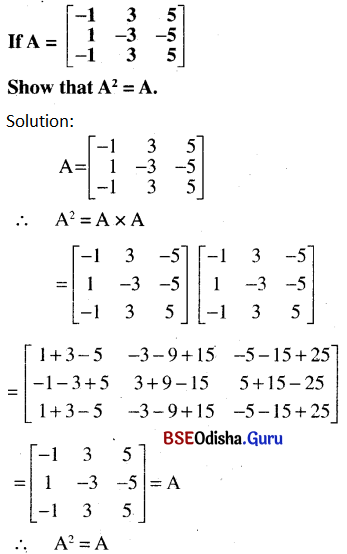Question 36.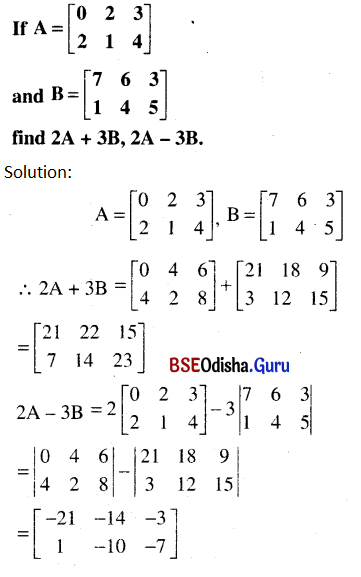Question 37.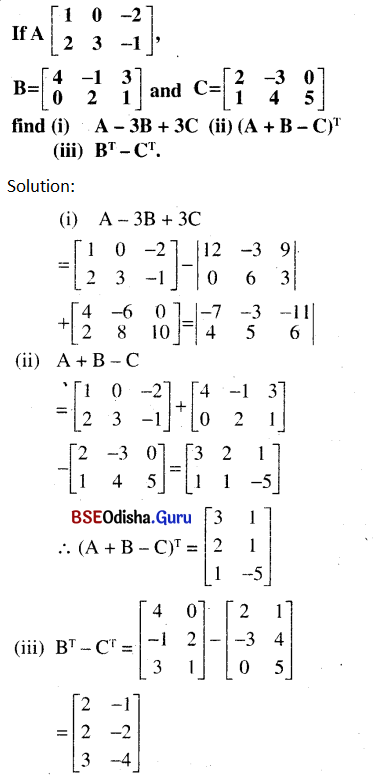Question 38.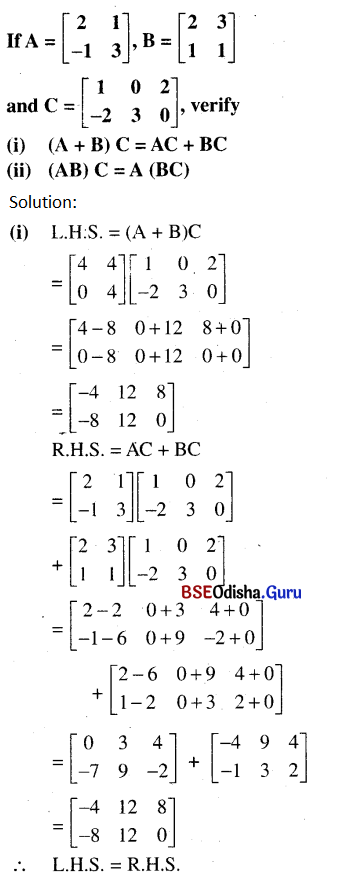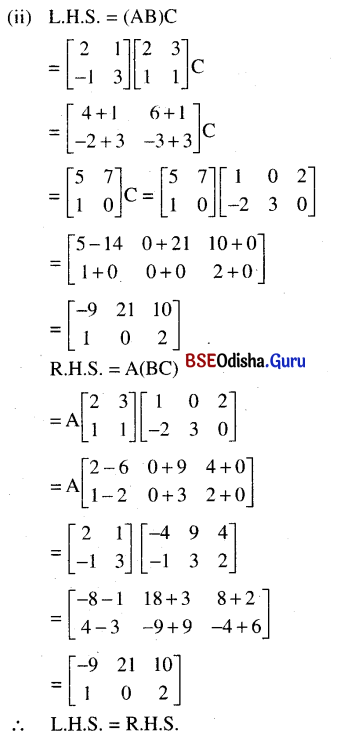Question 39.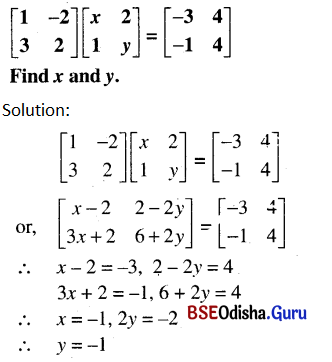Question 40.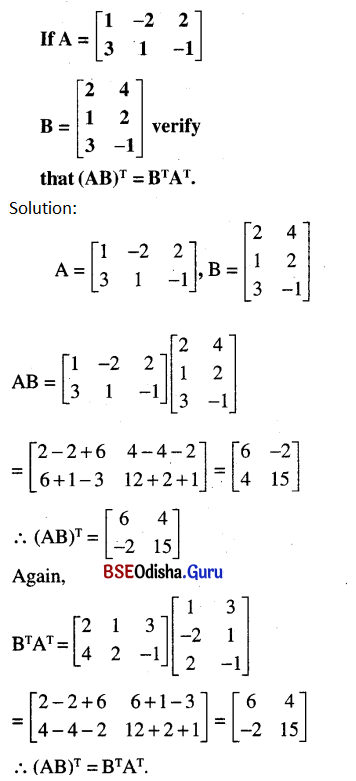Question 41.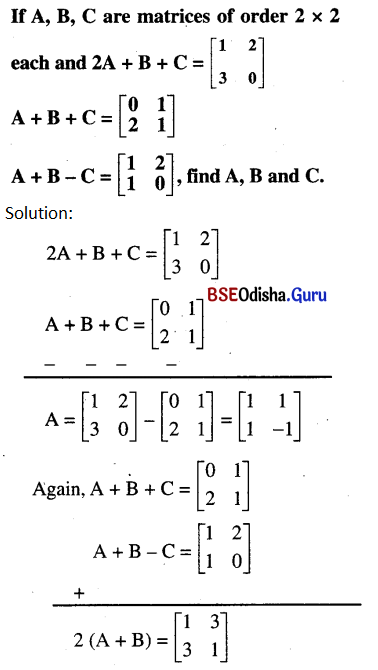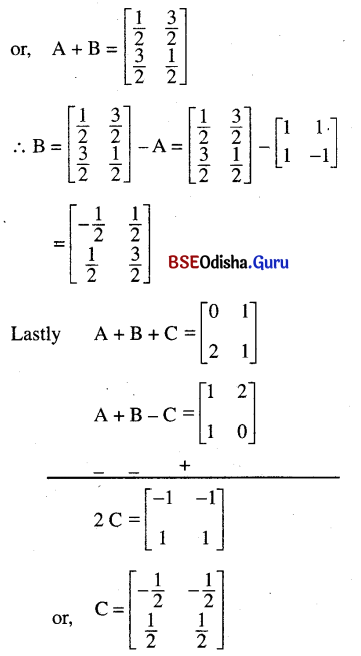Question 42.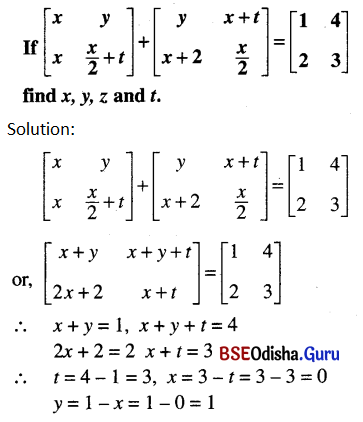Question 43.

 Men Women Children Family A → 4 6 2 Family B → 2 2 4
 Family B Calory Proteins Men 2400 45 Women 1900 55 Children 1800 33

Solution:
The given informations can be written in matrix form as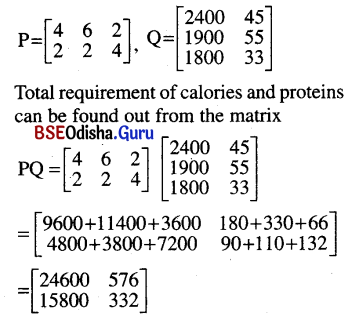∴ Calory requirements for families A and B are 24600 and 15800 respectively and protein requirements are 576 gm and 332 gm respectively.Question 44.
Let the investment in first fund = ₹x and in the second fund is ₹(50000-x)
Investment matrix A=[x  50000-x]⇒ 300000 – x = 278000
⇒ x = 22000
∴ He invests ₹22000 in first bond and ₹28000 in the second bond.